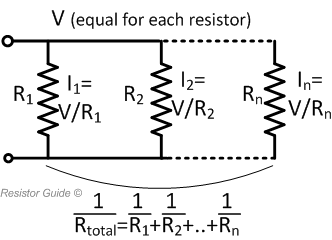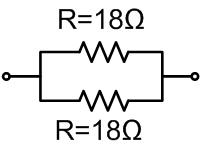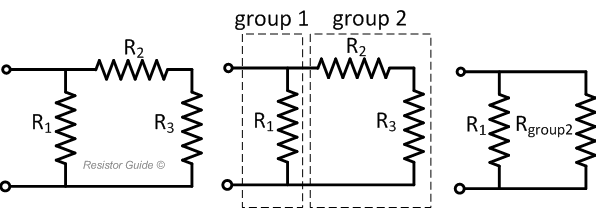# Resistors in Parallel

## How to Calculate the Equivalent Resistance for Resistors in Parallel?Resistors are often connected in series or parallel to create more complex networks. An example of resistors connected in parallel is shown in the figure above. The voltage across resistors in parallel is the same for each resistor. The current, however, is inversely proportional to the resistance of each individual resistor. The equivalent resistance of several resistors in parallel is given by:

$$\frac{1}{R_{eq}} = \sum_{i=1}^{n} \frac{1}{R_i} = \frac{1}{R_1} + \frac{1}{R_2} + \dots + \frac{1}{R_n}$$

The current through each resistor is given by:

$$I_i = \frac{V}{R_i}$$

To quickly calculate the equivalent resistance value of two resistors in parallel, you can use the parallel resistor calculator.

## Example Parallel Resistance Calculation

A circuit designer needs to install a resistor with 9 Ω and can choose from any of the E-12 series of preferred values (..., 10, 12, 15, 18, 22, 27, 33, 39, 47, 56, 68, 82, ...). Unfortunately, the value of 9 Ω is not available in this series. He decides to connect two standard values in parallel to create an equivalent resistance of 9 Ω. The equivalent resistance value for 2 resistors in parallel is calculated with these steps:

$$\frac{1}{R_{eq}} = \frac{1}{R_1} + \frac{1}{R_2}$$

$$\frac{1}{R_{eq}} = \frac{R_2}{R_1 · R_2} + \frac{R_1}{R_1 · R_2}$$

$$\frac{1}{R_{eq}} = \frac{R_1 + R_2}{R_1 · R_2}$$

$$R_{eq} = \frac{R_1 · R_2}{R_1 + R_2}$$

The above equation shows that if R1 is equal to R2, then Req is half of the value of one of the two resistors. For a Req of 9 Ω, R1 and R2 should therefore have a value of 2 x 9 = 18 Ω. This happens to be a standard value from the E-series.

$$R_{eq} = \frac{R_1 · R_2}{R_1 + R_2} = \frac{18 · 18}{18 + 18} = 9 \Omega$$

As a solution, the designer connects two resistors of 18 Ω in parallel as shown in the following figure to create an equivalent 9 Ω resistance.## How to Solve a Network with Resistors in Parallel and Series

More complex resistor networks can often easily be solved by systematic grouping of resistors. In the left image below, three resistors are connected as shown. Resistors R2 and R3 are connected in series. They are in parallel with resistor R1. To solve the network, the resistors are separated in two groups as illustrated in the middle figure. Group 1 consists of only R1. Group 2 consists of R2 and R3.The equivalent resistance of group 2 is easily calculated by the sum of R2 and R3:

$$R_{group2} = R_2 + R_3$$

This then leads to the simplified circuit shown at the right figure with two resistors in parallel. The equivalent resistance value of this circuit is easily calculated:

$$\frac{1}{R_{eq}} = \frac{1}{R_1} + \frac{1}{R_{group2}}$$

$$R_{eq} = \frac{R_1 · R_{group2}}{R_1 + R_{group2}}$$

$$R_{eq} = \frac{R_1 · (R_2 + R_3)}{R_1 + R_2 + R_3}$$

## Solving More Complex Networks with Kirchhoff's Laws

With repeated application of these rules for calculating resistors in parallel and resistors in series, larger networks can be solved. For more complex networks, Kirchhoff's laws can also be used.